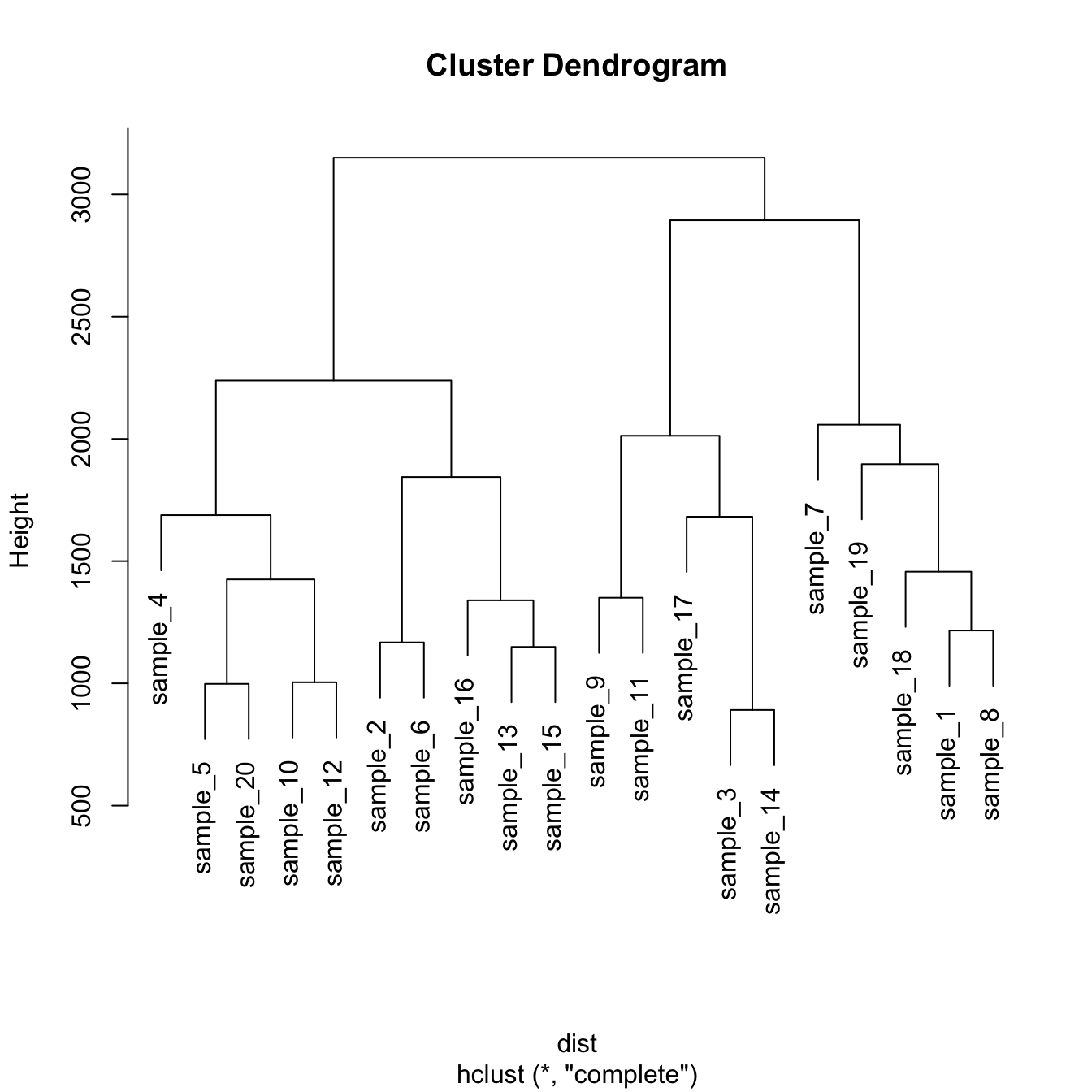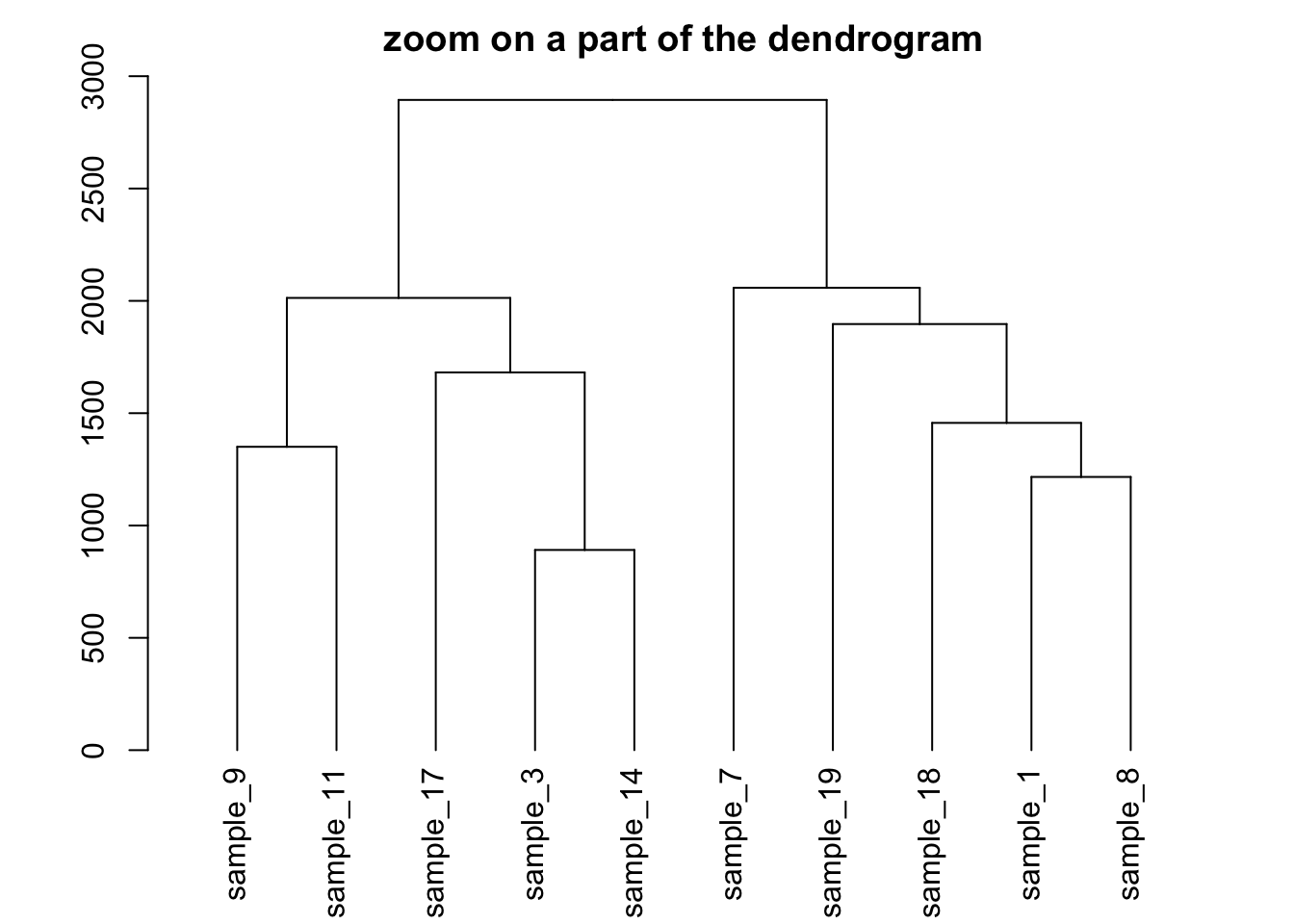# Most basic dendrogram for clustering with R

Clustering allows to group samples by similarity and can its result can be visualized as a dendrogram. This post describes a basic usage of the `hclust()` function and builds a dendrogram from its output.

# Most basic dendrogram with R

→ Input dataset is a `matrix` where each row is a sample, and each column is a variable. Keep in mind you can transpose a matrix using the `t()` function if needed.

→ Clustering is performed on a square matrix (sample x sample) that provides the distance between samples. It can be computed using the `dist()` or the `cor()` function depending on the question your asking

→ The `hclust()` function is used to perform the hierarchical clustering

→ Its output can be visualized directly with the `plot()` function. See possible customization.``````# Dataset
data <- matrix( sample(seq(1,2000),200), ncol = 10 )
rownames(data) <- paste0("sample_" , seq(1,20))
colnames(data) <- paste0("variable",seq(1,10))

# Euclidean distance
dist <- dist(data[ , c(4:8)] , diag=TRUE)

# Hierarchical Clustering with hclust
hc <- hclust(dist)

# Plot the result
plot(hc)``````

# Hierarchical clustering principle:

• Take distances between objects.
• Seek the smallest distance between 2 objects.
• Aggregate the 2 objects in a cluster.
• Replace them with their barycenter. → Again until having only one cluster containing every points.

There are several ways to calculate the distance between 2 clusters ( using the max between 2 points of the clusters, or the mean, or the min, or ward (default) ).

# Zoom on a group

It is possible to zoom on a specific part of the tree. Select the group of interest using the `[[..]]` operator:``````# store the dedrogram in an object
dhc <- as.dendrogram(hc)

# set the margin
par(mar=c(4,4,2,2))

# Plot the Second group
plot(dhc[] , main= "zoom on a part of the dendrogram")``````

Related chart types

## Contact

This document is a work by Yan Holtz. Any feedback is highly encouraged. You can fill an issue on Github, drop me a message on Twitter, or send an email pasting yan.holtz.data with gmail.com.